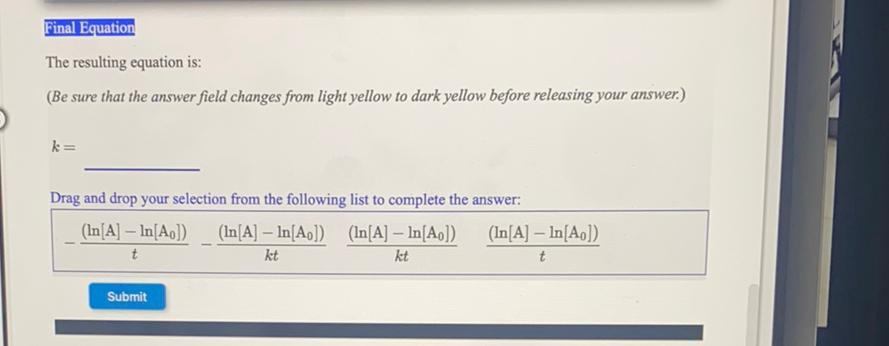Question:

# Final Equation The resulting equation is: (Be sure that the answer field changes from light yellow to dark yellow before releasiFinal Equation The resulting equation is: (Be sure that the answer field changes from light yellow to dark yellow before releasing your answer.) k= Drag and drop your selection from the following list to complete the answer: (In[A] - In[Ao] (In[A] - In[AD]) (In[A] - In[A]]) (In[A] - In[A]) t kt kt t Submit